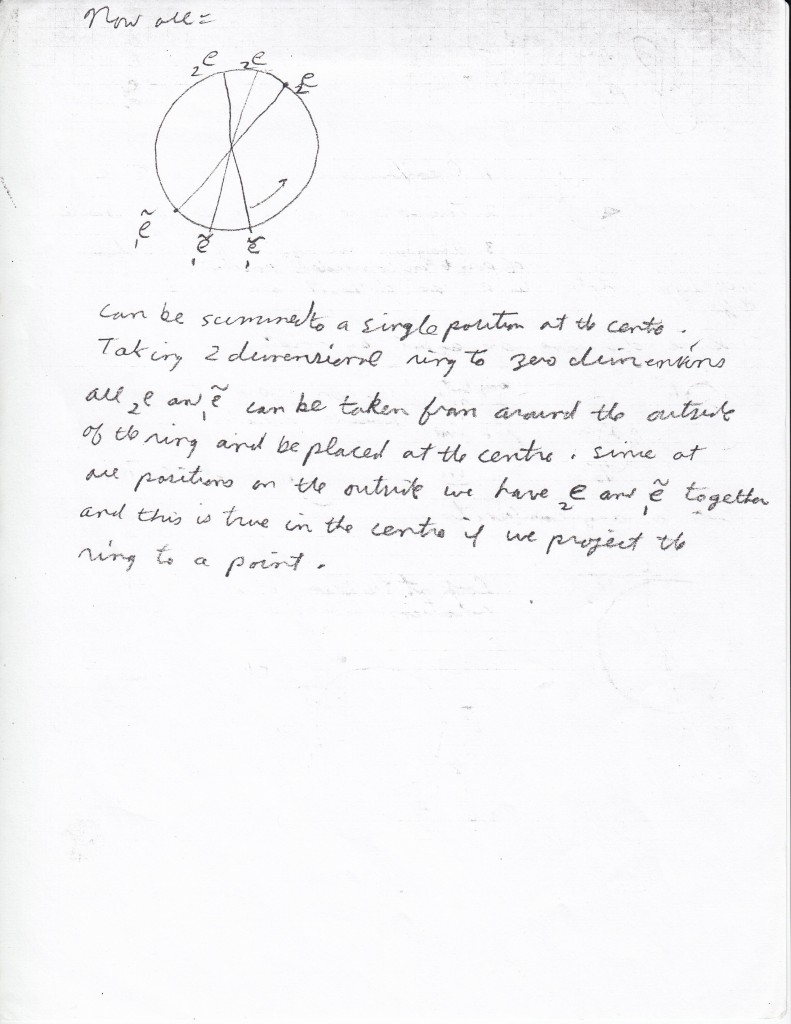# Interactive Online Tutoring Services

## May 1, 2016

### Deciding to add some notes

Below I have entered four pages of notes. I will add explanation to the diagrams over the next little while. Firstly, I will explain the notes using text and then I will add in the symbolism after that.

It has been seen from the idea of concept sharing that a place can have a place of places. So two places can have two places of places too. Then it should be possible to switch the two places and move in space. We need to be able to “move” as places.

Then instead of us actually being places we need to create a space we can climb into which acts like a point from the outside. Thus we need to find a way of turning a sphere into a point, on the outside. The first step then could be to find a way to do this two-dimensionally, that is find a way to map a circle to a point.

## April 29, 2016

### First page of notes

This note starts with the two locations A and B. But the space we are in is like two overlapping shadows, since we also have the further background of placements. That is, there is another coexisting plane where locations can be in other places, different from where they usually are relative to each other.

In order for a location to itself have another ‘location’ we need to have a coexisting plane which gives another ‘placement’ to any location. We give a new name to a ‘location’ of a location, since we can’t use the name ‘location’ again.

Let a plane of placements be created and coexist with a plane of locations. At the beginning a set of second co-ordinates is created. ((a,b),((a,b))). a and b are any real numbers. Each location (a,b) is at its usual place ((a,b)).

We can move locations in a closed loop or we can have a geometry where two or more locations share the same placement. This is called a joining. The parts of a joining are indistinguishable.

Then with A and B we can move A and B through other locations (creating a line of joinings) to a center where we have three locations and one placement.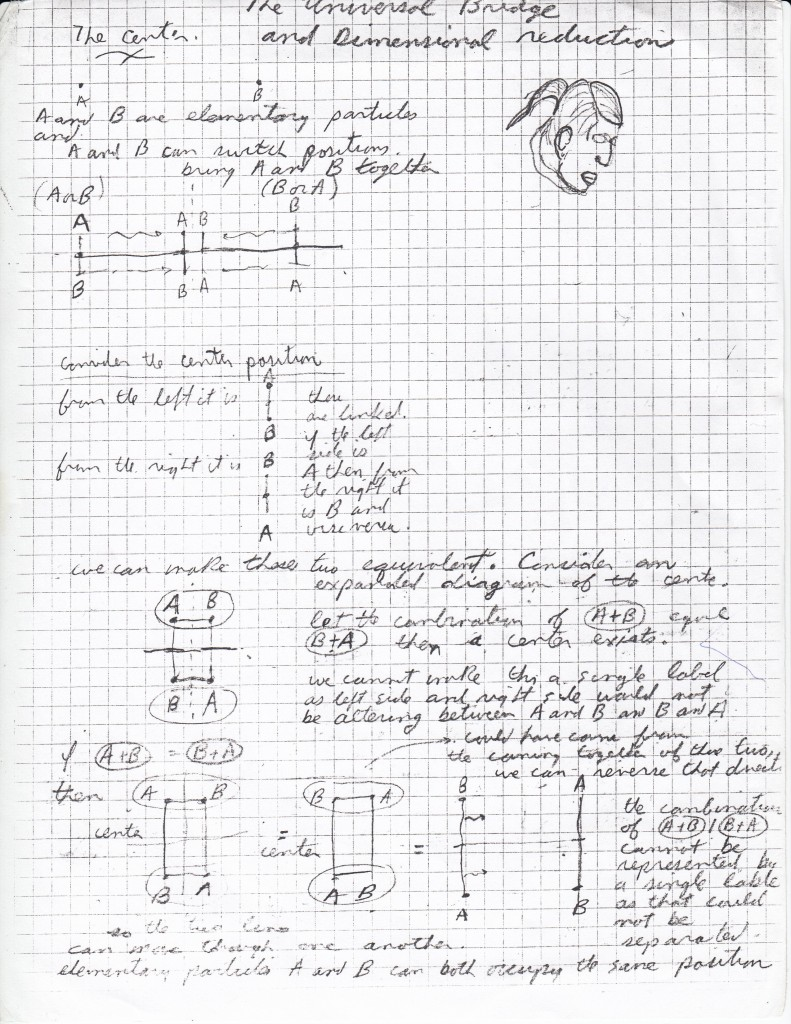### Second page of notes

This second note starts with a picture of a circle with radius r with a direction in the space modelled after the overlapping shadows.

The indicated locations can move through the three-dimensional space of placements and locations to eventually create a self-intersecting loop of locations. In order to do this a continuous series of joinings are gone through and a circle of voids, or just placements is left.

But we can also have the usual motion of a loop through space to give a self-intersecting loop. We can call this a move of the diagram, while in the other case we can call this a shift of the diagram. So the extra background gives us two different ways of altering the diagram.

What we can do then is move the diagram to create a self-intersecting loop in the usual sense. But I can reach this self-intersecting loop in two possible ways either by twisting through space one way or the other.

What I can do is create one diagram which covers both cases. If I place the center, as created in the first note at the self-intersection point of the self-intersecting  loop and then let the loop unloop itself but this time by shifting, not by moving. Then the multiple point b(1) has components e(1) and e(2) and e(1) and e(2) can switch positions. Then this models both twist cases.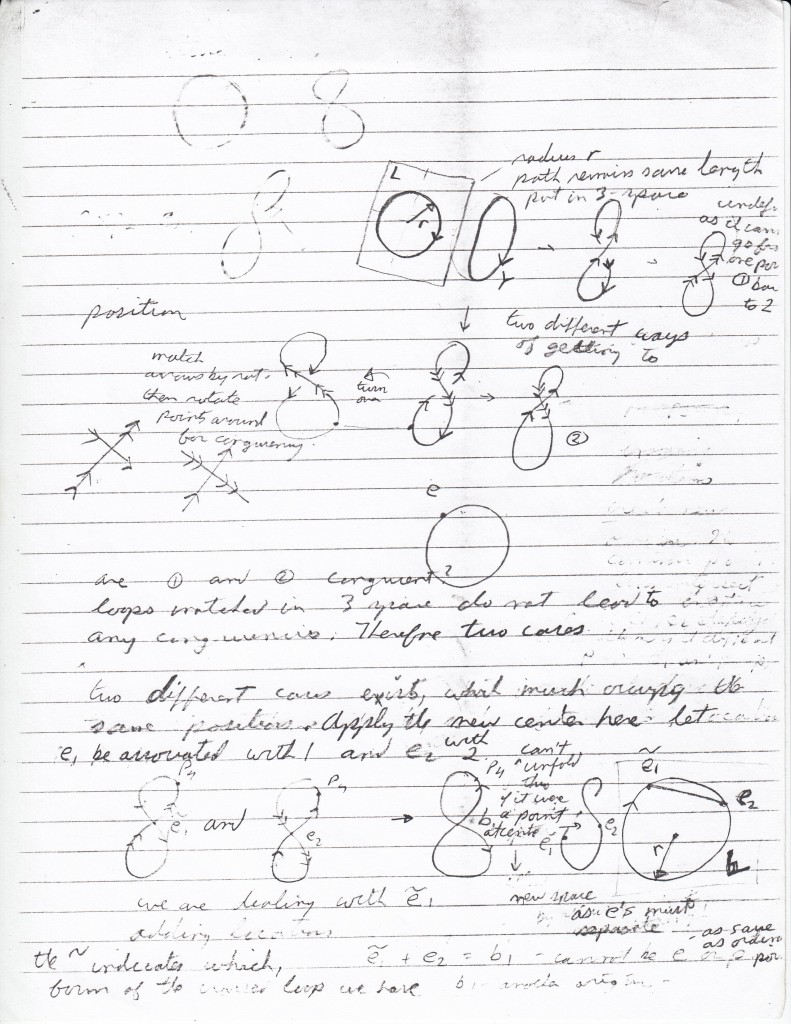### Third page of notes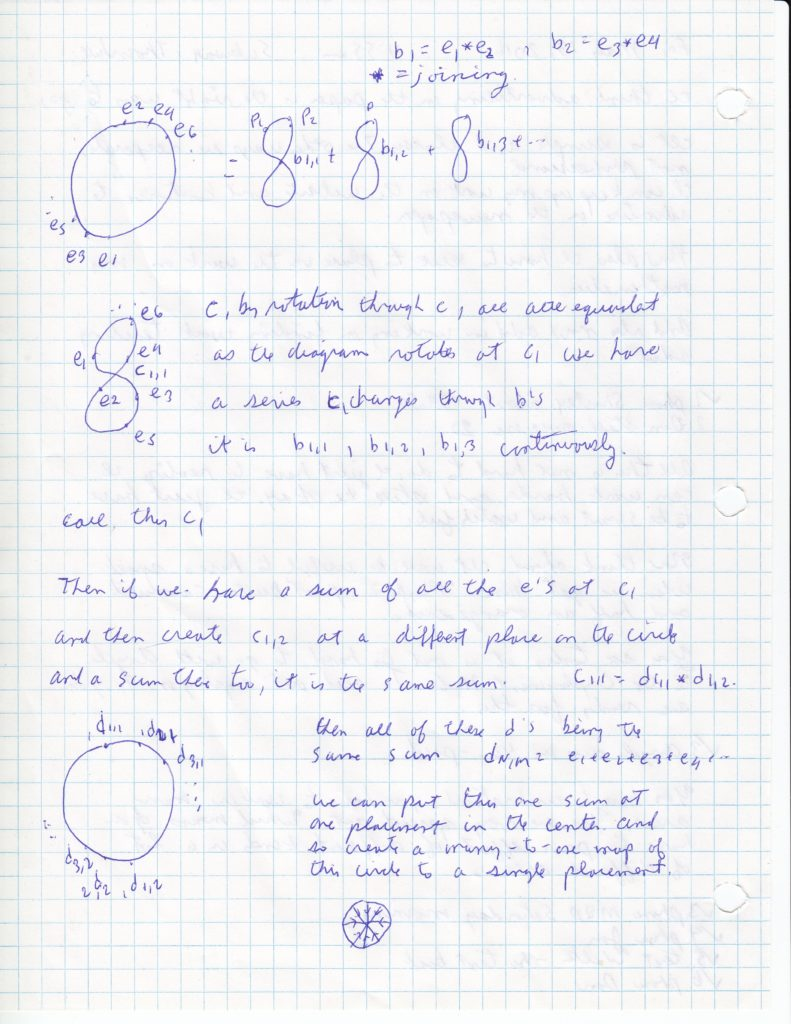The ideas of this page of notes follow from the previous page. b(1) is the joining of two p’s. But then we can also rotate the intersecting loop of locations around so that the locations of the loop occupy the same placements, but the locations change placements. That is the loop of locations rotates around and we define b(1) to be the same for all of these rotations.

The 1 refers to the self-intersecting loop being one for which half of the locations are above the intersection and half of the intersections are below the intersection. Call this now the placement b(1,1). b(1,1)=e(1)*e(2) where *=joining.

We can create a b(1,2) by shifting the self-intersecting loop slightly. Still half of the locations are above the intersection and half are below, yet we move the place where the loop intersects itself along just a little bit. Then also we can create b(1,3) and so on.

These loops can all be unfolded to each create a circle where we have the two e’s that create all of the different b’s. We can add all of these circles together by coincidence to get the first diagram on the left of the first page.

Then we can think of this as happening continuously too. So that we instead of moving a small distance to get from b(1,1) to b(1,2), we move in a continuous way.

Then next, we can think of this circle of e’s folded over once to intersect itself. Here we have c(1,1) but c must be a different idea than e. Let c be the idea that we sum all of the e’s in one place, once around the loop. Then we can have another c c(1,2) this will be another sum, in a slightly different order than c(1,1) but the sum will be the same.

Then if we unfold these and add, like we did previously we can obtain a circle of sums-all c’s. This one sum can also be placed at the center placement, so we can make a many to one map of a circle to a single placement.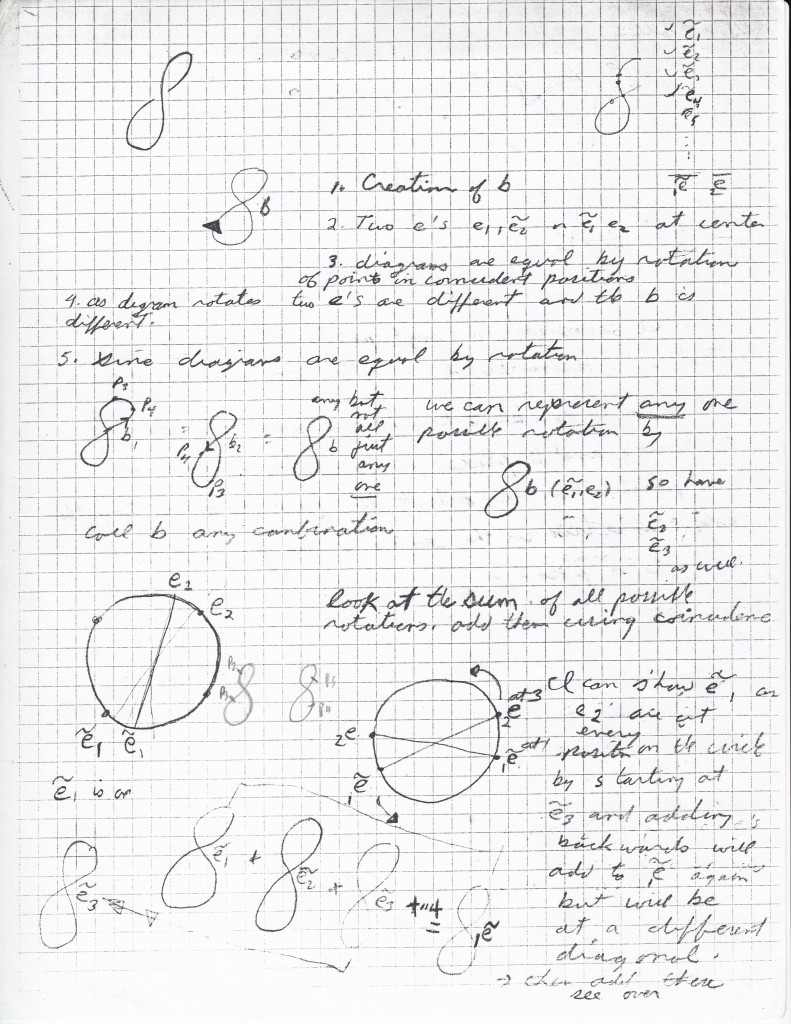### Forth page of notes

This forth page of notes is the conclusion that is come to from the previous three pages. The circle which is created with the sums around the circumference is moved in to a center placement and this is a many to one map. but the many refers to all of the same sums just added in a different order. This seems to be a way of changing the dimension of a ring from two dimensions to zero dimensions. Then also we could see each sum on the circumference of the circle as a little circle itself.